# seaborn.PairGrid¶

class `seaborn.``PairGrid`(data, hue=None, hue_order=None, palette=None, hue_kws=None, vars=None, x_vars=None, y_vars=None, corner=False, diag_sharey=True, height=2.5, aspect=1, layout_pad=0, despine=True, dropna=True, size=None)

Subplot grid for plotting pairwise relationships in a dataset.

This class maps each variable in a dataset onto a column and row in a grid of multiple axes. Different axes-level plotting functions can be used to draw bivariate plots in the upper and lower triangles, and the the marginal distribution of each variable can be shown on the diagonal.

It can also represent an additional level of conditionalization with the `hue` parameter, which plots different subsets of data in different colors. This uses color to resolve elements on a third dimension, but only draws subsets on top of each other and will not tailor the `hue` parameter for the specific visualization the way that axes-level functions that accept `hue` will.

`__init__`(self, data, hue=None, hue_order=None, palette=None, hue_kws=None, vars=None, x_vars=None, y_vars=None, corner=False, diag_sharey=True, height=2.5, aspect=1, layout_pad=0, despine=True, dropna=True, size=None)

Initialize the plot figure and PairGrid object.

Parameters

Tidy (long-form) dataframe where each column is a variable and each row is an observation.

huestring (variable name), optional

Variable in `data` to map plot aspects to different colors. This variable will be excluded from the default x and y variables.

hue_orderlist of strings

Order for the levels of the hue variable in the palette

palettedict or seaborn color palette

Set of colors for mapping the `hue` variable. If a dict, keys should be values in the `hue` variable.

hue_kwsdictionary of param -> list of values mapping

Other keyword arguments to insert into the plotting call to let other plot attributes vary across levels of the hue variable (e.g. the markers in a scatterplot).

varslist of variable names, optional

Variables within `data` to use, otherwise use every column with a numeric datatype.

{x, y}_varslists of variable names, optional

Variables within `data` to use separately for the rows and columns of the figure; i.e. to make a non-square plot.

cornerbool, optional

If True, don’t add axes to the upper (off-diagonal) triangle of the grid, making this a “corner” plot.

heightscalar, optional

Height (in inches) of each facet.

aspectscalar, optional

Aspect * height gives the width (in inches) of each facet.

Padding between axes; passed to `fig.tight_layout`.

despineboolean, optional

Remove the top and right spines from the plots.

dropnaboolean, optional

Drop missing values from the data before plotting.

`pairplot`

Easily drawing common uses of `PairGrid`.

`FacetGrid`

Subplot grid for plotting conditional relationships.

Examples

Draw a scatterplot for each pairwise relationship:

```>>> import matplotlib.pyplot as plt
>>> import seaborn as sns; sns.set()
>>> g = sns.PairGrid(iris)
>>> g = g.map(plt.scatter)
```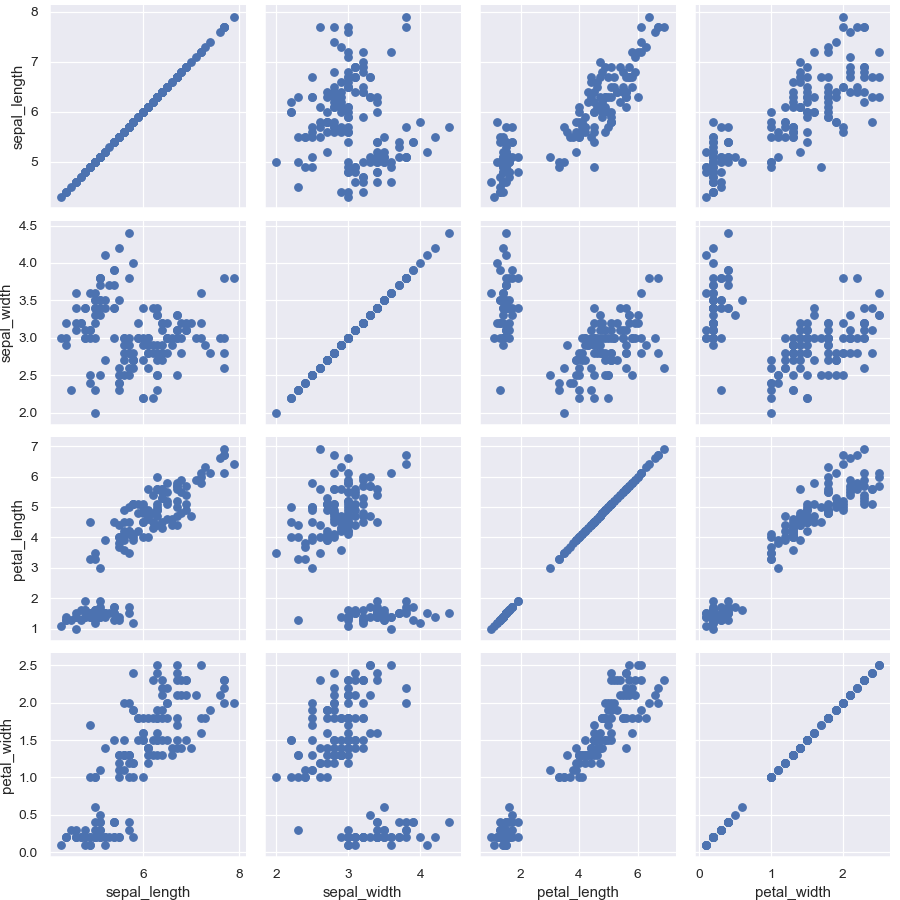Show a univariate distribution on the diagonal:

```>>> g = sns.PairGrid(iris)
>>> g = g.map_diag(plt.hist)
>>> g = g.map_offdiag(plt.scatter)
```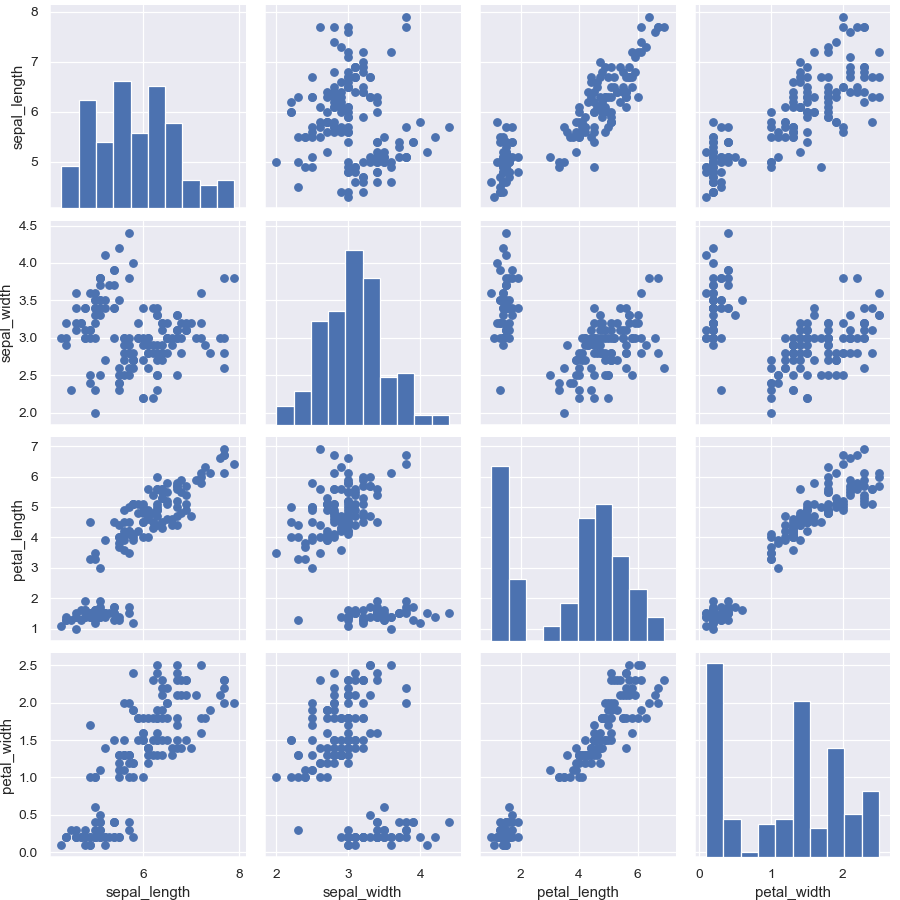(It’s not actually necessary to catch the return value every time, as it is the same object, but it makes it easier to deal with the doctests).

Color the points using a categorical variable:

```>>> g = sns.PairGrid(iris, hue="species")
>>> g = g.map_diag(plt.hist)
>>> g = g.map_offdiag(plt.scatter)
```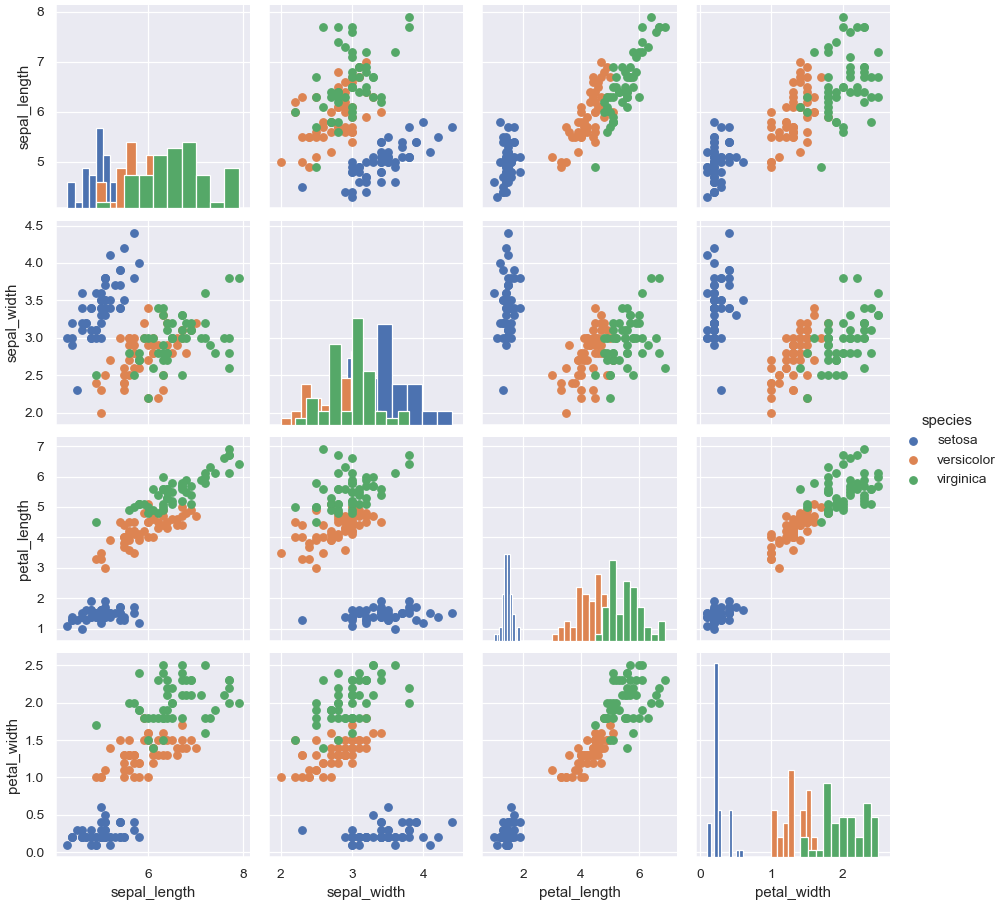Use a different style to show multiple histograms:

```>>> g = sns.PairGrid(iris, hue="species")
>>> g = g.map_diag(plt.hist, histtype="step", linewidth=3)
>>> g = g.map_offdiag(plt.scatter)
```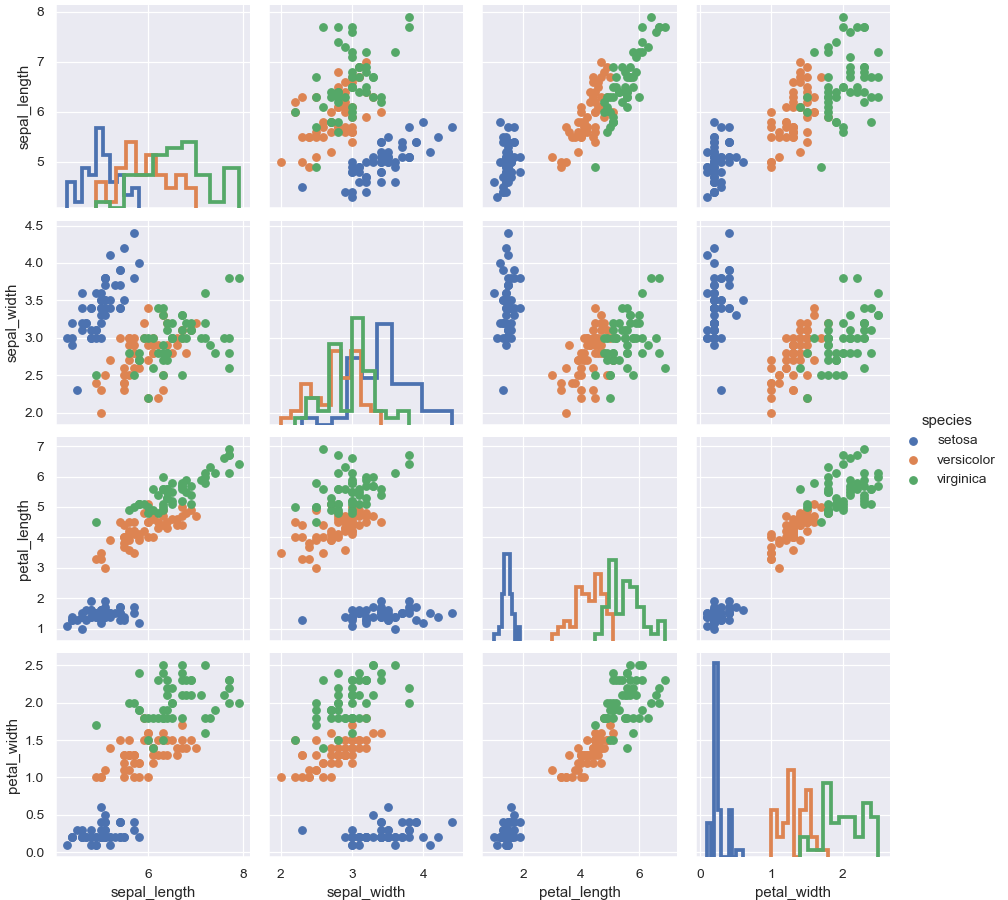Plot a subset of variables

```>>> g = sns.PairGrid(iris, vars=["sepal_length", "sepal_width"])
>>> g = g.map(plt.scatter)
```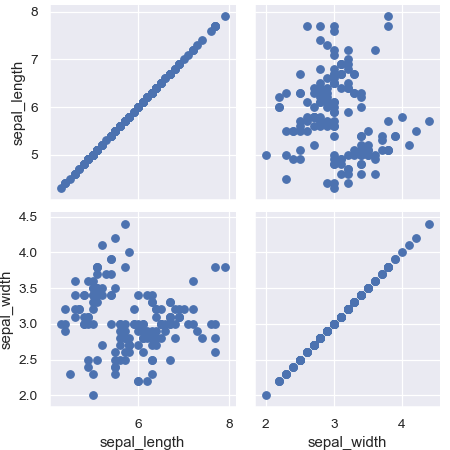Pass additional keyword arguments to the functions

```>>> g = sns.PairGrid(iris)
>>> g = g.map_diag(plt.hist, edgecolor="w")
>>> g = g.map_offdiag(plt.scatter, edgecolor="w", s=40)
```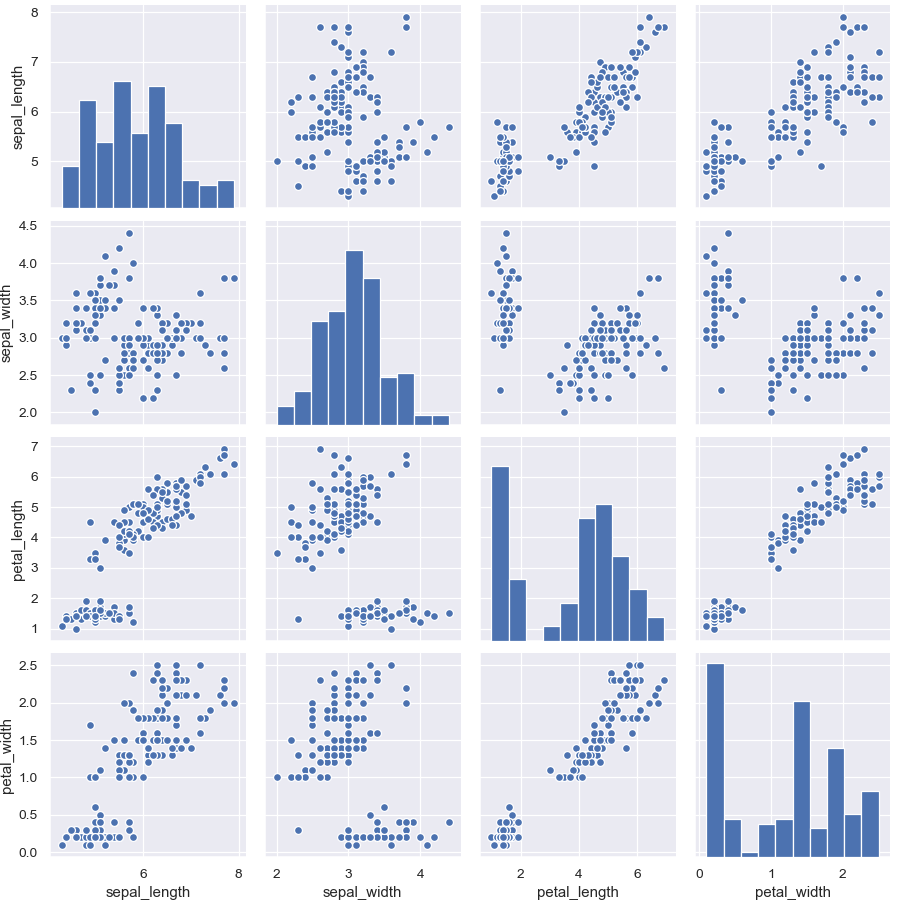Use different variables for the rows and columns:

```>>> g = sns.PairGrid(iris,
...                  x_vars=["sepal_length", "sepal_width"],
...                  y_vars=["petal_length", "petal_width"])
>>> g = g.map(plt.scatter)
```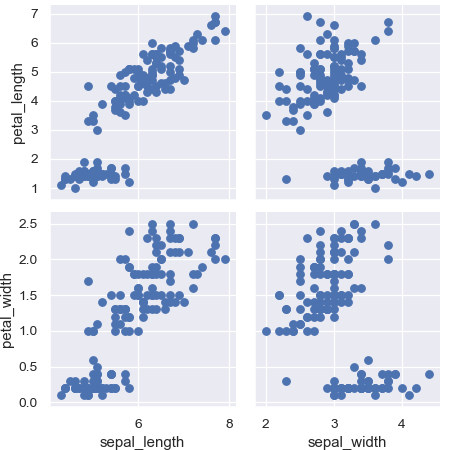Use different functions on the upper and lower triangles:

```>>> g = sns.PairGrid(iris)
>>> g = g.map_upper(sns.scatterplot)
>>> g = g.map_lower(sns.kdeplot, colors="C0")
>>> g = g.map_diag(sns.kdeplot, lw=2)
```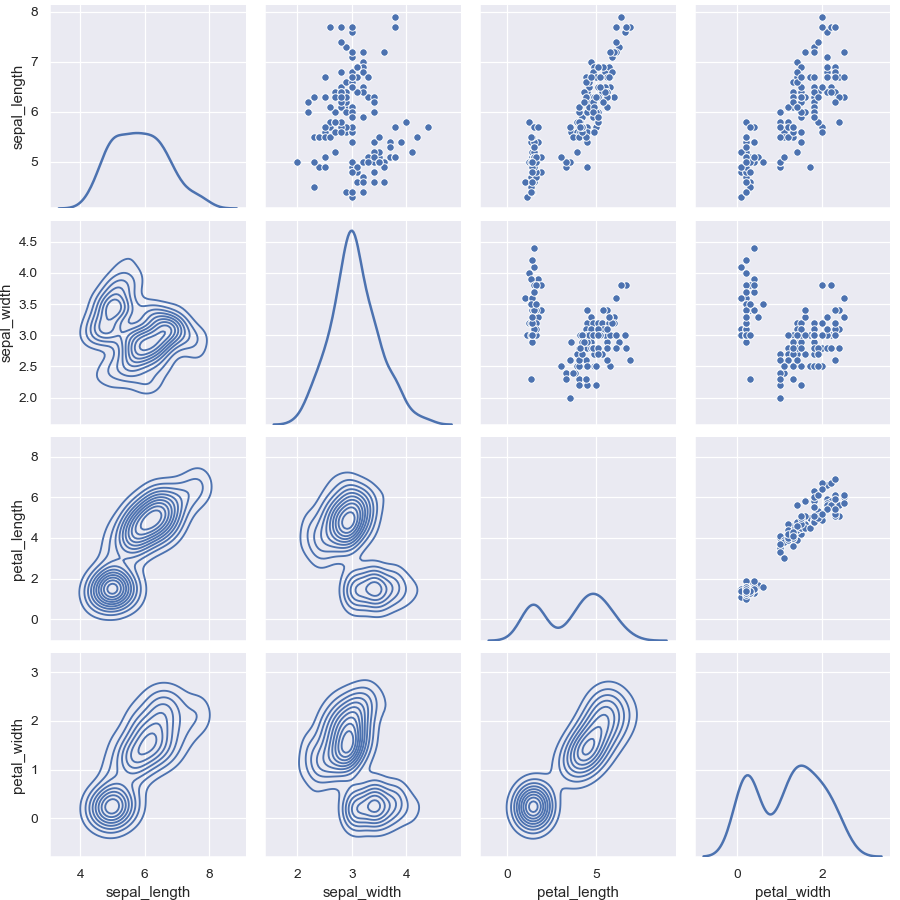Use different colors and markers for each categorical level:

```>>> g = sns.PairGrid(iris, hue="species", palette="Set2",
...                  hue_kws={"marker": ["o", "s", "D"]})
>>> g = g.map(sns.scatterplot, linewidths=1, edgecolor="w", s=40)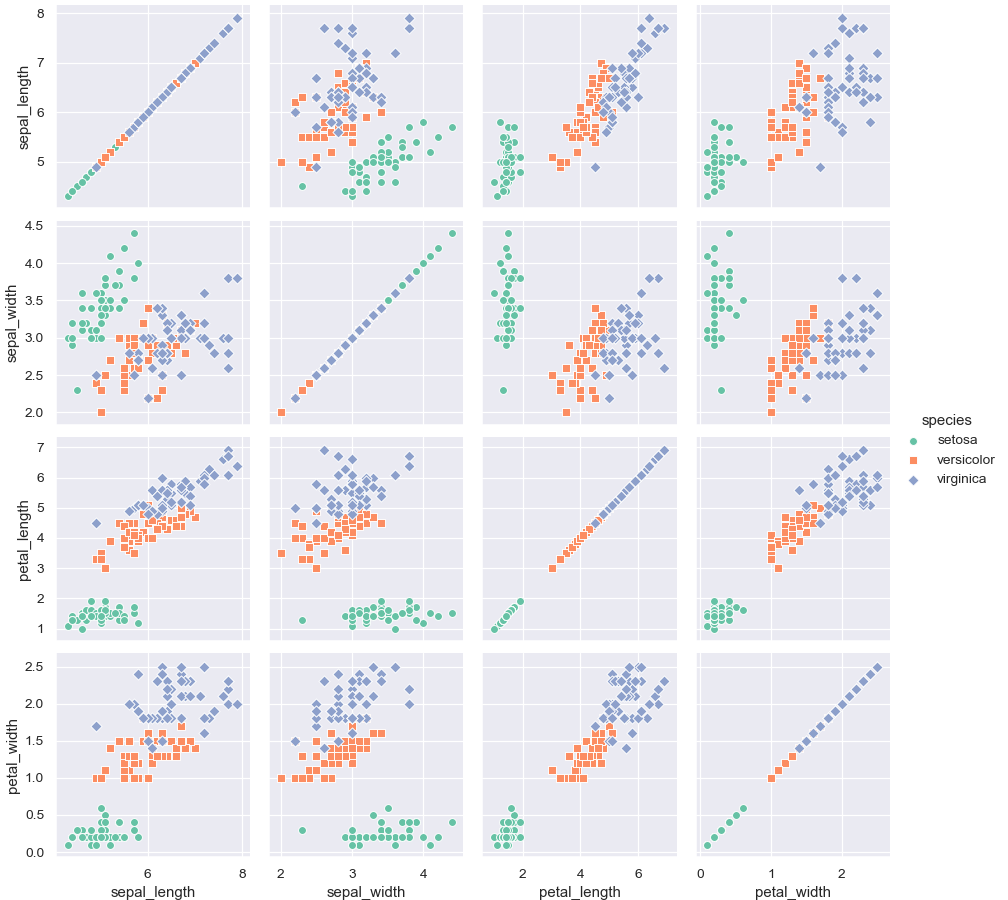`__init__`(self, data[, hue, hue_order, …]) Initialize the plot figure and PairGrid object. `add_legend`(self[, legend_data, title, …]) Draw a legend, maybe placing it outside axes and resizing the figure. `map`(self, func, \*\*kwargs) Plot with the same function in every subplot. `map_diag`(self, func, \*\*kwargs) Plot with a univariate function on each diagonal subplot. `map_lower`(self, func, \*\*kwargs) Plot with a bivariate function on the lower diagonal subplots. `map_offdiag`(self, func, \*\*kwargs) Plot with a bivariate function on the off-diagonal subplots. `map_upper`(self, func, \*\*kwargs) Plot with a bivariate function on the upper diagonal subplots. `savefig`(self, \*args, \*\*kwargs) Save the figure. `set`(self, \*\*kwargs) Set attributes on each subplot Axes.